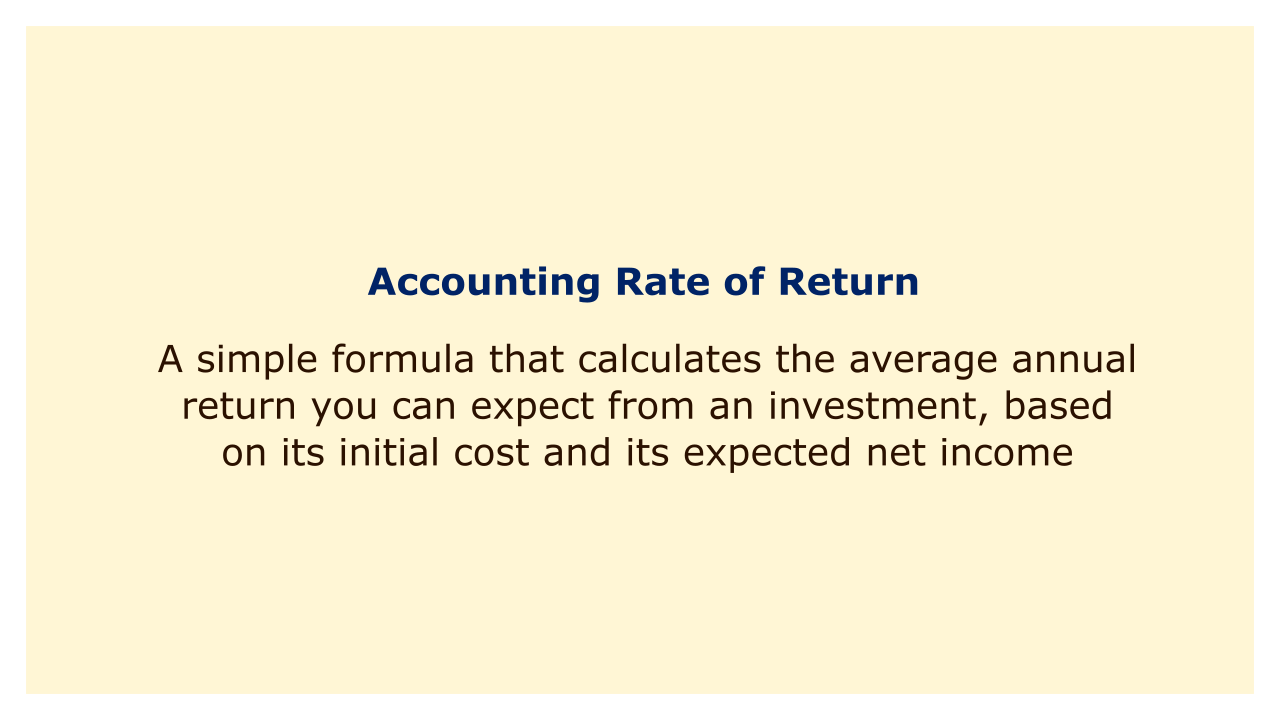# Accounting Rate of Return (ARR)Image: Moneybestpal.com

### The Accounting Rate of Return (ARR) is a simple formula that calculates the average annual return you can expect from an investment, based on its initial cost and its expected net income.

#### What is the Accounting Rate of Return?

The accounting rate of return is a ratio that compares the average annual profit of an investment to its initial cost. The formula for ARR is:

ARR = Average Annual Profit / Initial Investment

The average annual profit is the net income generated by the investment over its lifetime, divided by the number of years. The initial investment is the total amount of money spent to acquire or start the project.

For example, suppose you invest \$100,000 in a new machine that will generate \$20,000 of net income per year for 10 years. The ARR of this investment is:

ARR = (\$20,000 x 10) / \$100,000 / 10

ARR = 0.2 or 20%

This means that you can expect to earn 20% of your initial investment every year from this machine.

#### Why Use the Accounting Rate of Return?

The accounting rate of return is a useful tool for comparing different investment projects and choosing the most profitable one. It can help you answer questions like:
• How much return can I expect from this project?
• How does this project compare to other alternatives?
• Is this project worth investing in?

The ARR has some advantages over other methods of evaluating investments, such as:
• It is easy to calculate and understand.
• It uses accounting data that are readily available.
• It considers the entire income stream of the project, not just the cash flows.

#### What are the Limitations of the Accounting Rate of Return?

The accounting rate of return is not a perfect measure of profitability. It has some limitations that you should be aware of, such as:
• The time value of money is not taken into consideration, therefore it ignores the fact that money received today is worth more than money received in the future.
• It doesn't take the project's risk into consideration, therefore the return isn't adjusted for the illiquidity or fluctuation of the income stream.
• Because it does not take into consideration the various project lifespans, it can favor short-term projects over long-term ones.
Therefore, you should not rely solely on the ARR to make investment decisions. You should also use other methods, such as net present value (NPV), internal rate of return (IRR), or payback period (PP), to complement your analysis.
Tags